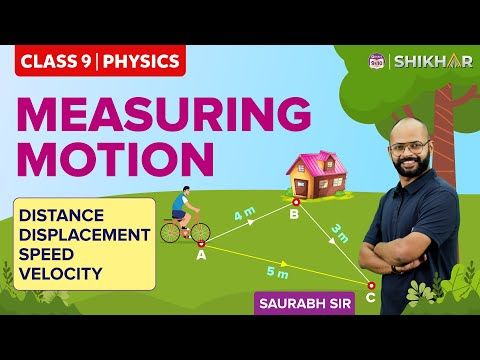# Difference between Distance and Displacement

Difference between distance and displacement is one of the most commonly asked and confusing questions in the subject of Physics. Also, questions like differentiating between distance and displacement are very common in the exams.To understand the differences, it is important to know their in-depth concepts thoroughly. By understanding the concepts of distance and displacement, understanding these differences will become easier. The difference between distance and displacement is tabulated below:

## Difference Between Distance and Displacement

 Sl. No. Differentiating Property Distance Displacement 1 Definition The complete length of the path between any two points is called distance. Displacement is the direct length between any two points when measured along the minimum path between them. 2 Denotation d s 3 Direction Consideration To calculate distance, the direction is not considered. To calculate displacement, the direction is taken into consideration. 4 Quantity Distance is a scalar quantity as it only depends upon the magnitude and not the direction. Displacement is a vector quantity as it depends upon both magnitude and direction. 5 Route Information Distance gives the detailed route information that is followed while travelling from one point to another. As displacement refers to the shortest path, it does not give complete information on the route. 6 Formula Speed × Time Velocity × Time 7 Possible Values The distance can only have positive values. Displacement can be positive, negative, and even zero. 8 Indication Distance is not indicated with an arrow. Displacement is always indicated with an arrow. 9 Measurement in Non-straight Path The distance can be measured along a non-straight path. Displacement can only be measured along a straight path. 10 Path Dependence Distance depends upon the path i.e. it changes according to the path taken. Displacement does not depend upon the path and it only depends upon the initial and final position of the body.

These are the main differences between distance and displacement that can help to distinguish these two thoroughly. These differences between distance and displacement, given in tabular form can also help students to retain the points in a better way.

Apart from knowing the comparison between distance and displacement, it is also suggested to know about their related concepts thoroughly and have a proper understanding of the basics. Check the related articles below to learn additional topics related to displacement and distance.

## Related Articles:

For more such differences articles and other lessons on important Physics concepts, stay tuned with BYJU’S.

Test your knowledge on Distance and displacement difference